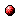# Max-Algebraic System Theory for Discrete Event Systems

Reference:
B. De Schutter, Max-Algebraic System Theory for Discrete Event Systems. PhD thesis, Faculty of Applied Sciences, K.U.Leuven, Leuven, Belgium, ISBN 90-5682-016-8, 331 pp., Feb. 1996.

Abstract:
Discrete event systems (DESs) are systems in which the state changes only at discrete points in time in response to the occurrence of particular events. Typical examples of DESs are: flexible manufacturing systems, telecommunication networks, parallel processing systems and traffic control systems. One of the frameworks that can be used to model and to analyze certain types of DESs is the max-plus algebra, which has maximization and addition as basic operations. In this thesis we develop tools for solving some fundamental problems in the max-algebraic system theory for DESs.
First we introduce a mathematical programming problem: the Extended Linear Complementarity Problem (ELCP). We develop an algorithm to find all the solutions of an ELCP.
We show that the problem of solving a system of multivariate max-algebraic polynomial equalities and inequalities is equivalent to an ELCP. This enables us to solve many other max-algebraic problems such as computing max-algebraic matrix factorizations, performing max-algebraic state space transformations, computing state space realizations of the impulse response of a max-linear time-invariant DES, computing max-algebraic singular value decompositions and QR decompositions, and so on.
We also study the max-algebraic characteristic polynomial and state space transformations for max-linear time-invariant DESs. Next we develop a method to solve the minimal state space realization problem for max-linear time-invariant DESs. First we use our results on the max-algebraic characteristic polynomial to develop a procedure to determine a lower bound for the minimal system order of a max-linear time-invariant DES. Then we show that the ELCP can be used to compute all fixed order partial state space realizations and all minimal state space realizations of the impulse response of a max-linear time-invariant DES.
Finally we prove the existence of max-algebraic analogues of two basic matrix decompositions from linear algebra: the singular value decomposition and the QR decomposition.

Downloads:PhD thesis: pdf file (1.72 MB)List of errata (as of November 30, 2008): pdf file (146 KB)
Note: More information on the pdf file format mentioned above can be found here.

Bibtex entry:

@phdthesis{DeS:96-02,
author={B. {D}e Schutter},
title={Max-Algebraic System Theory for Discrete Event Systems},
school={Faculty of Applied Sciences, K.U.Leuven},
address={Leuven, Belgium},
month=feb,
year={1996}
}

@misc{DeS:96-02-errata,
author={B. De Schutter},
title={Errata for the PhD thesis of Bart De Schutter ``Max-Algebraic System Theory for Discrete Event Systems''},
note={Last update: November 30, 2008.}
}

Go to the publications overview page.

This page is maintained by Bart De Schutter. Last update: August 12, 2020.